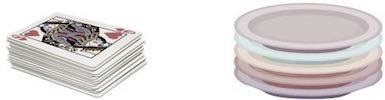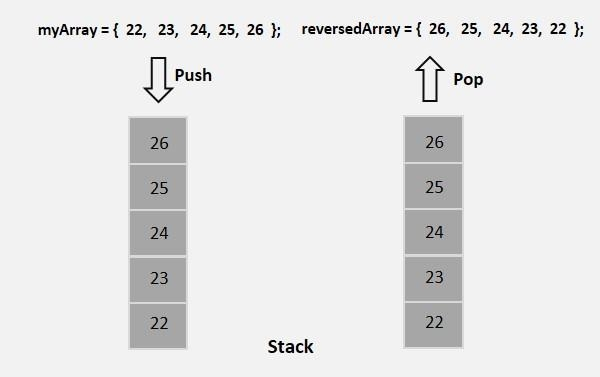# How to reverse the elements of an array using stack in java?

Stack is an Abstract Data Type (ADT), commonly used in most programming languages. It is named stack as it behaves like a real-world stack, for example – a deck of cards or a pile of plates, etc.A stack is first in first out, it has two main operations push and pop. Push inserts data in to it and pop retrieves data from it.

To reverse an array using stack initially push all elements in to the stack using the push() method then, retrieve them back using the pop() method into another array.## Example

Live Demo

import java.util.Arrays;
import java.util.Stack;

public class ReversinArrayUsingStack {
public static void main(String args[]) {
Stack<Integer> stack = new Stack<Integer>();
int[] myArray = {23, 93, 56, 92, 39};
int size = myArray.length;
for(int i=0; i<size; i++) {
stack.push(myArray[i]);
}
int[] reverseArray = new int[size];
for(int i=0; i<size; i++) {
reverseArray[i] = stack.pop();
}
System.out.println("Reversed array is ::"+Arrays.toString(reverseArray));
}
}

## Output

Reversed array is ::[39, 92, 56, 93, 23]